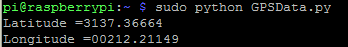Page 1 of 1

### Convert NMEA sentence Lat and Lon to Decimal Degrees

Posted: Tue Feb 21, 2017 11:33 pm
Hi freinds,

i have this python code, using NMEA sentence (\$GPRMC)

i need to convert the latitude ang longitude to decimal degress, The details are in this link : http://notinthemanual.blogspot.com/2008 ... de-to.html

Code: Select all

``````import serial

ser = serial.Serial('/dev/ttyUSB0', 9600,timeout=1)

pos1 = x.find("\$GPRMC")
pos2 = x.find("\n", pos1)
loc  = x[pos1:pos2]
data = loc.split(',')
if data == 'V':
print 'No location found'
else
print "Latitude =" + data
print "Longitude =" + data``````
This is the results when i run the code :### Re: Convert NMEA sentence Lat and Lon to Decimal Degrees

Posted: Wed Feb 22, 2017 12:32 am
So...

Lat : 3137.36664 becomes 31 degrees and 37.26664 seconds = 31 + 37.36664/60 = 31.6227773
Lon : 00212.21149 becomes 2 degrees and 12.21149 seconds = 2 + 12.21149/60 = 2.20352483

So as latitude is in format DDSS.SSSSS
DD = int(float(Lat)/100) = int(3137.36664/100) = int(31.3736664) = 31
SS = float(lat) - DD * 100 = 3137.36664 - 31 * 100 = 3137.36664 - 3100 = 37.36664

LatDec = DD + SS/60 = 31 + 37.36664/60 = 31 + 0.6227773333333333 = 31.6227773333333333

Don't forget that data will be your North/South indicator, i.e. "S" or "N".
If this is "S" you need to negate your LatDec value.

The processing for the longitude will be very similar. This time data will be your East/West indicator. So negate if "W".

### Re: Convert NMEA sentence Lat and Lon to Decimal Degrees

Posted: Wed Feb 22, 2017 12:44 am
Perfect and very detailed reply, thank you very much
Mortimer wrote:So...

Lat : 3137.36664 becomes 31 degrees and 37.26664 seconds = 31 + 37.36664/60 = 31.6227773
Lon : 00212.21149 becomes 2 degrees and 12.21149 seconds = 2 + 12.21149/60 = 2.20352483

So as latitude is in format DDSS.SSSSS
DD = int(float(Lat)/100) = int(3137.36664/100) = int(31.3736664) = 31
SS = float(lat) - DD * 100 = 3137.36664 - 31 * 100 = 3137.36664 - 3100 = 37.36664

LatDec = DD + SS/60 = 31 + 37.36664/60 = 31 + 0.6227773333333333 = 31.6227773333333333

Don't forget that data will be your North/South indicator, i.e. "S" or "N".
If this is "S" you need to negate your LatDec value.

The processing for the longitude will be very similar. This time data will be your East/West indicator. So negate if "W".

### Re: Convert NMEA sentence Lat and Lon to Decimal Degrees

Posted: Thu Apr 11, 2019 7:03 pm
Just to be clear, I believe that wherever "seconds" was written, what was intended was minutes.

### Re: Convert NMEA sentence Lat and Lon to Decimal Degrees

Posted: Sun Apr 14, 2019 10:52 am
My word! How silly of me.
Amazing it stood for so long undetected!

Corrected (for what it's worth):

So...

Lat : 3137.36664 becomes 31 degrees and 37.26664 seconds = 31 + 37.36664/60 = 31.6227773
Lon : 00212.21149 becomes 2 degrees and 12.21149 seconds = 2 + 12.21149/60 = 2.20352483

So as latitude is in format DDMM.MMMMM
DD = int(float(Lat)/100) = int(3137.36664/100) = int(31.3736664) = 31
MM = float(lat) - DD * 100 = 3137.36664 - 31 * 100 = 3137.36664 - 3100 = 37.36664

LatDec = DD + MM/60 = 31 + 37.36664/60 = 31 + 0.6227773333333333 = 31.6227773333333333

Don't forget that data will be your North/South indicator, i.e. "S" or "N".
If this is "S" you need to negate your LatDec value.

The processing for the longitude will be very similar. This time data will be your East/West indicator. So negate if "W".

Still at least the sums worked out OK!